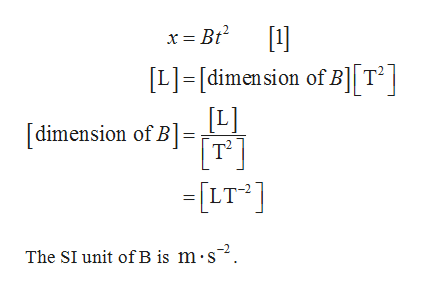# (a) Suppose the displacement of an object is related to timeaccording to the expression x = Bt2. What are the dimensionsof B? (b) A displacement is related to time as x= A sin (2πft),where A and f are constants. Find the dimensions of A. Hint:A trigonometric function appearing in an equation must bedimensionless.

Question
1 views

(a) Suppose the displacement of an object is related to time
according to the expression x = Bt2. What are the dimensions
of B? (b) A displacement is related to time as x= A sin (2πft),
where A and f are constants. Find the dimensions of A. Hint:
A trigonometric function appearing in an equation must be
dimensionless.

check_circle

Step 1

(a) The dimension of B given...help_outlineImage Transcriptionclosex = Bt² [L]=[dimension of B][T°] [L] [T] =[LT ] [dimension of B]= The SI unit ofB is m·s². fullscreen

### Want to see the full answer?

See Solution

#### Want to see this answer and more?

Solutions are written by subject experts who are available 24/7. Questions are typically answered within 1 hour.*

See Solution
*Response times may vary by subject and question.
Tagged in

### Physics